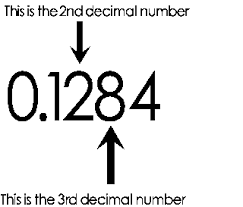# Forge

Sort by:
Most Popular
results for tag
"decimal"by
David Sousa
1.0.0  (O10)
Latest release
29 Mar 2019
decimal
input
mobileRandomNumberFromList
by
Shwetha M
The Component can be used to generate Random Number(integer/decimal) From List and also it can be used to get list of Random Number(integer/decimal) From List.
1.0.0  (O10)
Latest release
20 Dec 2017
random
List
integer
decimal
NumberDecimalCalcs
by
Pedro Vargues
This extension does simple maths operations (sum/subtract/multiply/divide) with 2 given integer/decimal numbers (saved in text) and returns the result with more than 8 decimals, if that's the case (in text).
1.0.0  (O11)
Latest release
2 May 2019
calculations
decimal
numbersAutonumeric
by
The best and more complete component to mask yours fields of decimal, numeric, currency, percent and much more. Check all possible configuration at http://autonumeric.org/configurator *No conflict with Jquery Mask Plugin*
1.0.1  (O11)
Latest release
5 Apr 2020
Currency
decimal
input
International
numeric
percentRoundingWithGivenPrecision.xif
by
Vivek Singh
It rounds off decimal to a specified precision . Example 35.00449--&gt;35.01(If we want to round off 2 decimal places) 35.00449 will be rounded off to 35.01 if you give input with precision of 2 ,currently I had the requirement but couldn't find any function that rounds off with given precision , this is for .NET platform Input inputDecimalString(string) Precision(Integer) OutPut OutputDecimalString(String)